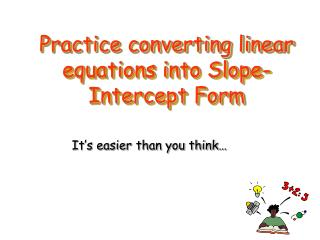DownloadDownload PresentationPractice converting linear equations into Slope-Intercept Form

# Practice converting linear equations into Slope-Intercept Form

Download Presentation## Practice converting linear equations into Slope-Intercept Form

- - - - - - - - - - - - - - - - - - - - - - - - - - - E N D - - - - - - - - - - - - - - - - - - - - - - - - - - -
##### Presentation Transcript

1. Practice converting linear equations into Slope-Intercept Form It’s easier than you think…

2. Slope intercept form is: y = mx + b Our main goal is to get the y alone on one side of the equation

3. Convert Into Slope-Intercept Form (divide both sides by 2 to get y alone) (now simplify all fractions) 2 1

4. Intercept slope Now look at the equation below…… When an equation is in slope-intercept form: y = mx + b 2 What is the slope? ____________ 1 What is the intercept? ____________

5. Now look at the graph of the line. y = 2x + 1 Step 1: Look at the y-intercept and plot where the graphs cross the “y” axis. Step 2: Use the slope (rise/run) to determine the next point and plot. Step 3: Draw a line through both points. Be sure to extend pass point and put arrow at both ends.

6. *** Easy *** Convert to Slope-Intercept Form: 5y = 10x + 15 (divide both sides by 5 to get y alone) (now simplify all fractions) y = 2x + 3 BRAVO!!

7. *** Now Try this Convert to Slope-Intercept Form*** -3y = -9x - 12 Step 1: divide both sides by -3 to get y alone Step 2: Simplify all fractions Step 3: Write your equation in y = mx + b What is the slope? ____________ What is the intercept? ____________

8. -3-3 -3y = -9x - 12 -3-3 -3 *** Check Your Answer *** -3y = -9x - 12 (divide both sides by -3 to get y alone) (now simplify all fractions) y = 3x + 4 Wow, you’re good at this!! Slope = 3 Intercept = 4

9. *** medium *** Convert to Slope-Intercept Form: 21x – 7y =14 (subtract both sides by 21x) (now divide both sides by -7) -7y = -21x + 14 (simplify all fractions) y = 3x – 2

10. *** Now Try this Convert to Slope-Intercept Form*** 2y + 26 = -6x Step 1: Subtract both sides by 26 Step 2: Divide both sides by 2 to get y by itself Step 3: Simplify all fractions What is the slope? ____________ What is the intercept? ____________

11. *** Check Your Answer*** 2y + 26 = -6x (subtract both sides by 26) (now divide both sides by 2 2y = -6x - 26 (simplify all fractions) y = -3x - 13 You are a math wizard!

12. Graphing from the slope-intercept form • Graph ½ x + 2 • Locate the y-intercept on the graph and plot the point.

13. Plotting the second pointusing the slope ½ • Move up 1 and right 2 and plot the second point.

14. Plotting the slope

15. Drawing the line • Now use a straight edge and connect the two points. • You can check your work, by choosing any point located on the line and substituting the point into the equation. The equation will be true no matter what point you chose.

16. Applying slope-intercept to graphing inequalities Write the equation in slope-intercept form just like you have learned in the previous slides. Graph the equation as if it contains an equal sign.

17. Graph the inequality 2x-3y>6 • First write the equation in slope-intercept form • 2x-3y>6 -2x -2xsubtract 2x on both sides -3y > -2x + 6 -3 -3 -3divide each term by -3

18. y <2 x + 2 reverse the sign because you divided by 3 a negative number. The y-intercept is 2 and the slope is 2 3 Use this information to graph the inequality This is easy for you!

19. Graph the y-intercept y< 2 x + 2 3

20. y < 2 x + 2 3 • Use the slope to graph the second point.

21. y < 2 x + 2 3 • Before connecting the two points with a line, first look at the inequality symbol. In this case I must use a dashed line.

22. y < 2 x + 2 3 • The final step is to shade the half –plane. • two choices- • look at the inequality symbol and understand that < means the half-plane below the boundary line or

23. y < 2 x + 2 3 pick and point and substitute it into the equation. If the point makes the equation true then that is the half-plane that I shade. If it is false I shade the other side of the half plane. Way to go. You have the hang of this now

24. y < 2 x + 2 3 • In this equation I shade below the half plane. You’re a math Genius!!

25. Practice the problems on your handout! • I know you will do well